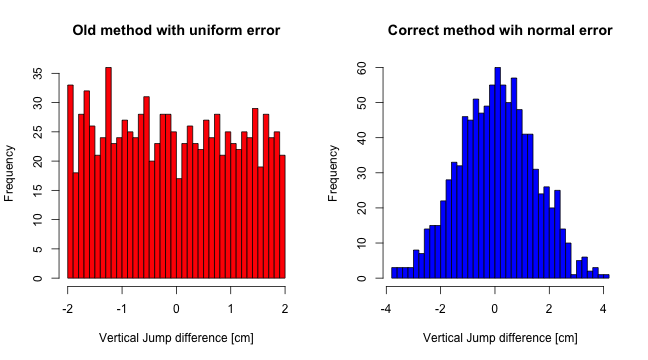## Tuesday, November 12, 2013

### Quick correction in simulating Typical Error

Quick correction in simulating Typical Error

# Quick correction in simulating Typical Error

I am awaiting review and opinions/critiques from statistics wizzard Will Hopkins for my How to visualize test change scores for coaches, but even on his first look he pointed to one small error I did in simulating typical error for vertical jump. It is not huge error, but it is an error in representing typical error in measurement.

### What I did wrong?

This is what I did wrong (for this example I will generate 1000 athletes/samples)

``````# Load library for random names generation for athlete
library(randomNames)

# Simulate vertical jump scores for 1000 players, with an average of 55cm
# and SD of 6cm We are going to repeat the assessment on two days in the
# same conditionins and add some 'noise'
set.seed(505)

reliabilityTest1CM_old <- rnorm(1000, mean = 55, sd = 6)
reliabilityTest2CM_old <- reliabilityTest1CM_old + runif(1000, min = -2, max = 2)

# Let's name those 1000 athletes
reliabilityPlayerNames_old <- randomNames(1000)

# Put the data in data frame
reliabilityData_old <- data.frame(Athlete = reliabilityPlayerNames_old, Test1 = reliabilityTest1CM_old,
Test2 = reliabilityTest2CM_old)

# Calcualte the differences between tests
reliabilityData_old <- within(reliabilityData_old, Diff <- Test2 - Test1)
``````

Here are our first 10 athletes from the table

Athlete Test1 Test2 Diff
1 O'Connor, Joshua 48.27 49.04 0.76
2 Matthews, Tisa 47.31 46.94 -0.37
3 Obi, Victoria 42.76 42.88 0.12
4 Leal, Alexandra 49.37 48.09 -1.28
5 Salazar, Ana 51.94 53.61 1.68
6 Nunez-Medina, Makeyla 52.52 53.17 0.65
7 Shin, Nicholas 49.54 49.81 0.28
8 Smith, Bruce 55.84 57.57 1.73
9 Bayarsaikhan, Gabriel 58.39 58.10 -0.29
10 Yarangsy, Miles 52.41 52.13 -0.27

What I did wrong is that I have added uniform noise to the first test

reliabilityTest1CM_old <- rnorm(1000, mean=55, sd=6)

reliabilityTest2CM_old <- reliabilityTest1CM_old + runif(1000, min=-2, max=2)

What I should have done to simulate this more methodically and correctly is to create real test scores and add normal “noise” (TE) to BOTH of them. Here is the right code

``````set.seed(505)

# Create the 'REAL' scores in vertical jump with mean=55 and SD=6 for 1000
# athletes
realVerticalJumpCM <- rnorm(1000, mean = 55, sd = 6)

# Now add 'noise' (TE) to both reliability tests. In this case noise has
# mean=0 and SD=1. If mean of the TE would be >0 then that would be a
# constant error.

reliabilityTest1CM <- realVerticalJumpCM + rnorm(1000, mean = 0, sd = 1)
reliabilityTest2CM <- realVerticalJumpCM + rnorm(1000, mean = 0, sd = 1)

# Let's name those 1000 athletes
reliabilityPlayerNames <- randomNames(1000)

# Put the data in data frame
reliabilityData <- data.frame(Athlete = reliabilityPlayerNames, Test1 = reliabilityTest1CM,
Test2 = reliabilityTest2CM)

# Calcualte the differences between tests
reliabilityData <- within(reliabilityData, Diff <- Test2 - Test1)
``````
Athlete Test1 Test2 Diff
1 Martinez, Kuan-Hsuen 48.77 47.65 -1.12
2 Earvin, Colton 47.38 46.84 -0.54
3 Vigil, Analee 44.16 43.63 -0.53
4 Bruce, Victoria 49.55 49.44 -0.11
5 Slechter, Joshua 51.76 52.26 0.50
6 Kaeser, Lnay 52.35 54.24 1.89
7 Shibles, Kevin 50.82 49.11 -1.70
8 Russell, Jonathan 54.21 56.85 2.64
9 Chavez, Selena 58.96 58.21 -0.75
10 Saldivar, Ebonnie 51.09 52.33 1.24

Here is the histogram of difference scores between test1 and test2 in both methods

``````par(mfrow = c(1, 2))

hist(reliabilityData_old\$Diff, 30, col = "red", main = "Old method with uniform error",
xlab = "Vertical Jump difference [cm]")

hist(reliabilityData\$Diff, 30, col = "blue", main = "Correct method wih normal error",
xlab = "Vertical Jump difference [cm]")
``````Let's calculate TE for both simulations. I need to copy paste our function for TE calculus first.

``````typicalError <- function(data, test1 = "Test1", test2 = "Test2", na.rm = TRUE) {
# Remove the missing values
if (na.rm)
data <- na.omit(data)

# Calculate the difference from named collums
diff <- data[[test2]] - data[[test1]]

return(sd(diff)/sqrt(2))
}

# Calculate Typical Errors for both simulations
TE_old <- typicalError(reliabilityData_old)
TE_correct <- typicalError(reliabilityData)

cat("Simulation #1 with uniform error\nTE=", round(TE_old, 3), "\n")
``````
``````## Simulation #1 with uniform error
## TE= 0.822
``````
``````cat("\nSimulation #2 with normal error\nTE=", round(TE_correct, 3), "\n")
``````
``````##
## Simulation #2 with normal error
## TE= 0.987
``````

As you can see, TE in correct method of simulation (TE=0.987) is VERY close to SD=1 of our normally distributed noise we have added to the “real” vertical jump.

I hope I haven't made any errors now… But Will will notice :)